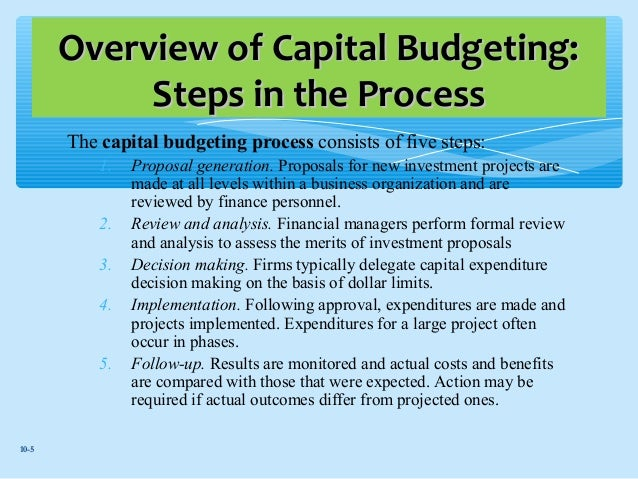# Basics Steps in Capital BudgetingConceptually, the capital budgeting process involves six logical steps.  First, the cost of the project must be determined.  This is similar to finding the price that must be paid for a stock or bond.  Next, management must estimate the expected cash flows from the project, including the value of the asset at a specified terminal date.  This is similar to estimating the future dividend or interest payment stream on a stock or bond.   Third, the riskiness of projected cash flows must be estimated.   To do this, management needs information about the probability distributions of the cash flows. Fourth,  given  the  riskiness  of projected  cash  flows  and  the cost  of funds  under prevailing economic conditions as reflected by the riskless rate, RF, the firm must determine the appropriate discount rate, or cost of capital, at which the project=s cash flows are to be discounted.   This is equivalent to finding the required rate of return on a stock or a bond investment.  Fifth, expected cash flows are converted to a present value basis to obtain a clear estimate of the investment project=s value to the firm.  This is equivalent to finding the present value of expected future dividends or interest  plus  principal  payment.    Finally,  the present  value of the expected  cash inflows is compared with the required outlay, or cost, of the project.  If the present value of cash flows derived from a project exceed the cost of the investment, the project should be accepted.  Otherwise, the project should be rejected.